<< Previous    1...   13  14    16  17  ...19    Next >>

#15 How the sacred number 528 demarcates space-time from all higher levels of reality

We pointed out on page 2 that the four highest Trees in the 7-tree mapping the physical plane represent the four etheric subplanes spoken about in Theosophy and that their inner form embodies one of the structural parameters of the basic unit of etheric matter. There are 504 yods in the inner form of each overlapping Tree of Life outside the root edge of the (7+7) enfolded polygons that are intrinsic to it because none of them are yods that belong also to its outer form: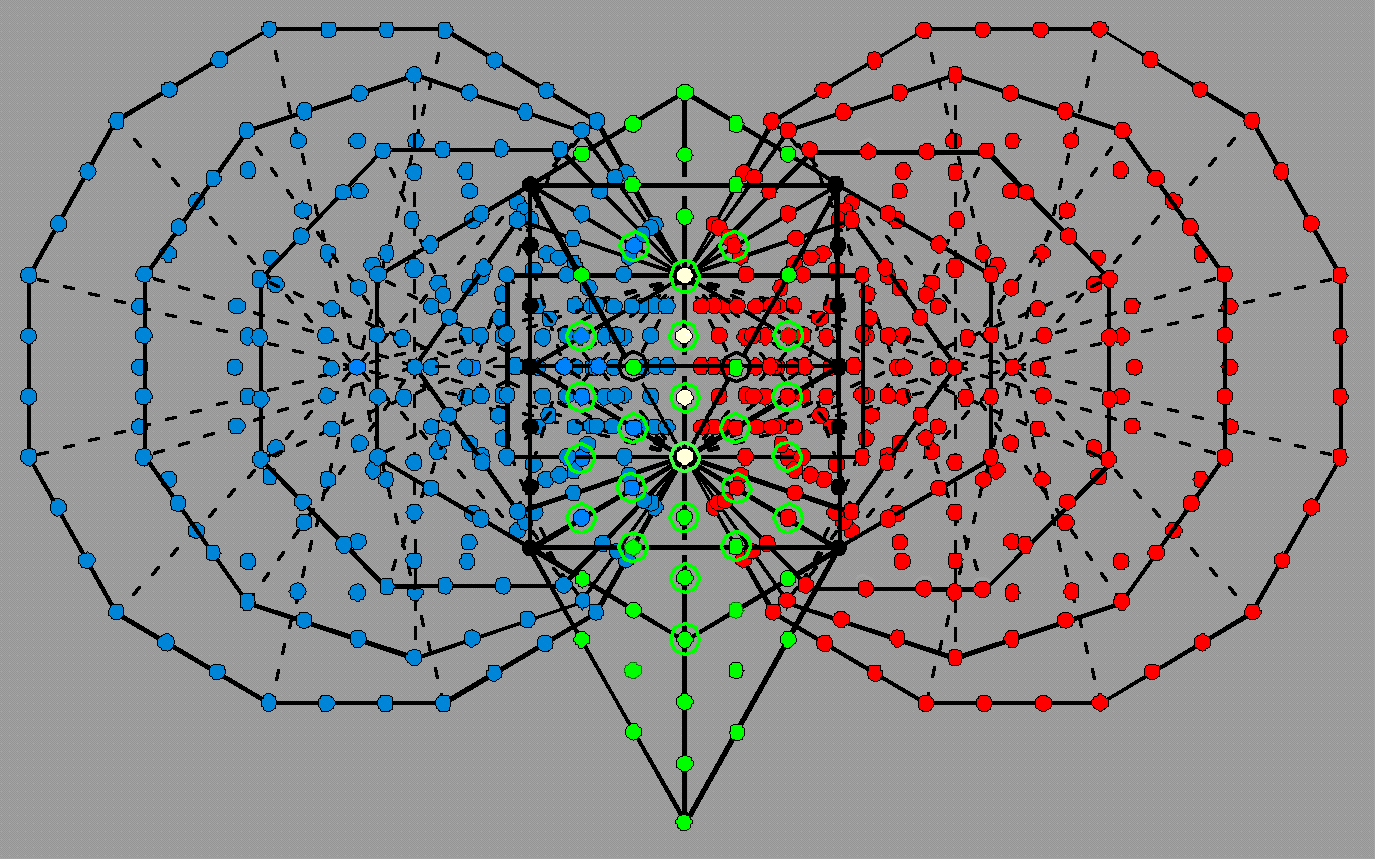The 504 red & blue yods outside the 4 white yods in the root edge belong solely to the inner Tree of Life; the (8+8=16) black yods are shared with the outer Tree of Life.

The inner form of the four uppermost Trees in the 7-tree contains (4×504=2016) intrinsic yods. They symbolise the 2016 turns in each helical whorl of the E8-singlet state of the E8×E8′ heterotic superstring. It must be emphasized that the structural parameter 2016 has been derived from totally independent considerations, namely, by hypothesizing that the 144 Polyhedron in the Polyhedral Tree of Life embodies in its geometrical composition the number of turns in a whorl of this particle, just as the other polyhedron — the disdyakis triacontahedron — embodies in its geometry the number of turns in each whorl of the UPA. This seems a reasonable extrapolation to make in view of the status (established earlier) of these two polyhedra as the polyhedral counterparts of, respectively, the branches and trunk of the Tree of Life. The deduction made from this hypothesis is that the former number = 6/5×1680 = 2016, where 6/5 is the ratio (72/60) of the numbers of vertices surrounding the axes of the 144 Polyhedron and disdyakis triacontahedron. We saw previously in this section that this ratio is the ratio of the numbers of sides of triangles in the branches of the Tree of Life and in its trunk, as well as the ratio of the number (144) of yods inside the sides of the seven enfolded polygons constructed from tetractyses and the number (120) of yods lining them: 144/120 = 6/5. The independent origin of the number 2016 makes it highly significant that 2016 factorises as 4×504. If we had examined the inner form of, say, three Trees, this would have been incompatible with the factorisation: 2016 = 3×672 because there are 524 yods in the inner form of each Tree, i.e., less than the number needed, so that the number cannot quantify any part of the inner form of just three Trees, whilst the next possible factorisation: 2016 = 6×336, although numerically possible, is excluded becauase it requires Trees that map either:

1. the two lowest subplanes of the next higher plane to be involved in the make-up of a particle of matter that belongs solely to the physical plane (albeit its etheric subplanes), or
2. six subplanes of this plane, thereby including at least some (if not all) of what Theosophy calls the three "dense physical" subplanes.

In either case, subplanes other that the etheric ones would have to contribute to the structural parameter of a particle belonging only to the former, and this clearly makes no sense. Only four subplanes/Trees are compatible in a meaningful way with any of the possible factorisations of 2016, and that is, precisely, the number of etheric subplanes spoken of in Theosophy. It confirms that the particle discovered by Ron Cowen with his micro-psi vision is, indeed, a particle of etheric matter. To deny this is to believe that it is pure coincidence that an independently determined number is embodied in the inner form of the very number of Trees of Life that is theoretically required in order to be consistent with the nature of the particle. But how can it be more "scientific" to choose the less plausible option? Personal disbelief towards the paranormal cannot be permitted to trump common sense.

As 504 = 336×3/2, where 336 is the number of turns per revolution of a whorl, this number is the number of circularly polarised oscillations in 1½ revolutions. As, according to Cowen, a whorl of the basic unit of etheric matter makes six revolutions (three in an outer spiral, three in a narrower spiral around its axis), we see that each of the four Trees mapping an etheric subplane corresponds to a quarter of a whorl. There have to be six revolutions of each whorl because 6 = 4×1½ and 2016 = 6×336. Each half of the inner form of a Tree contains 252 intrinsic yods outside its root edge that symbolise the number of oscillations in 1/8th of a whorl of this particle, i.e., in 6/8 (= 3/4) of a revolution. This implied eight-fold division into two sets of four is highly significant because it indicates that each whorl conforms to the archetypal pattern symbolised in Taoist cosmology by the eight trigrams. Indeed, this pattern applies to each revolution of a helical whorl because it comprises 336 turns along which are spread eight E8′ gauge charges, 42 turns per charge: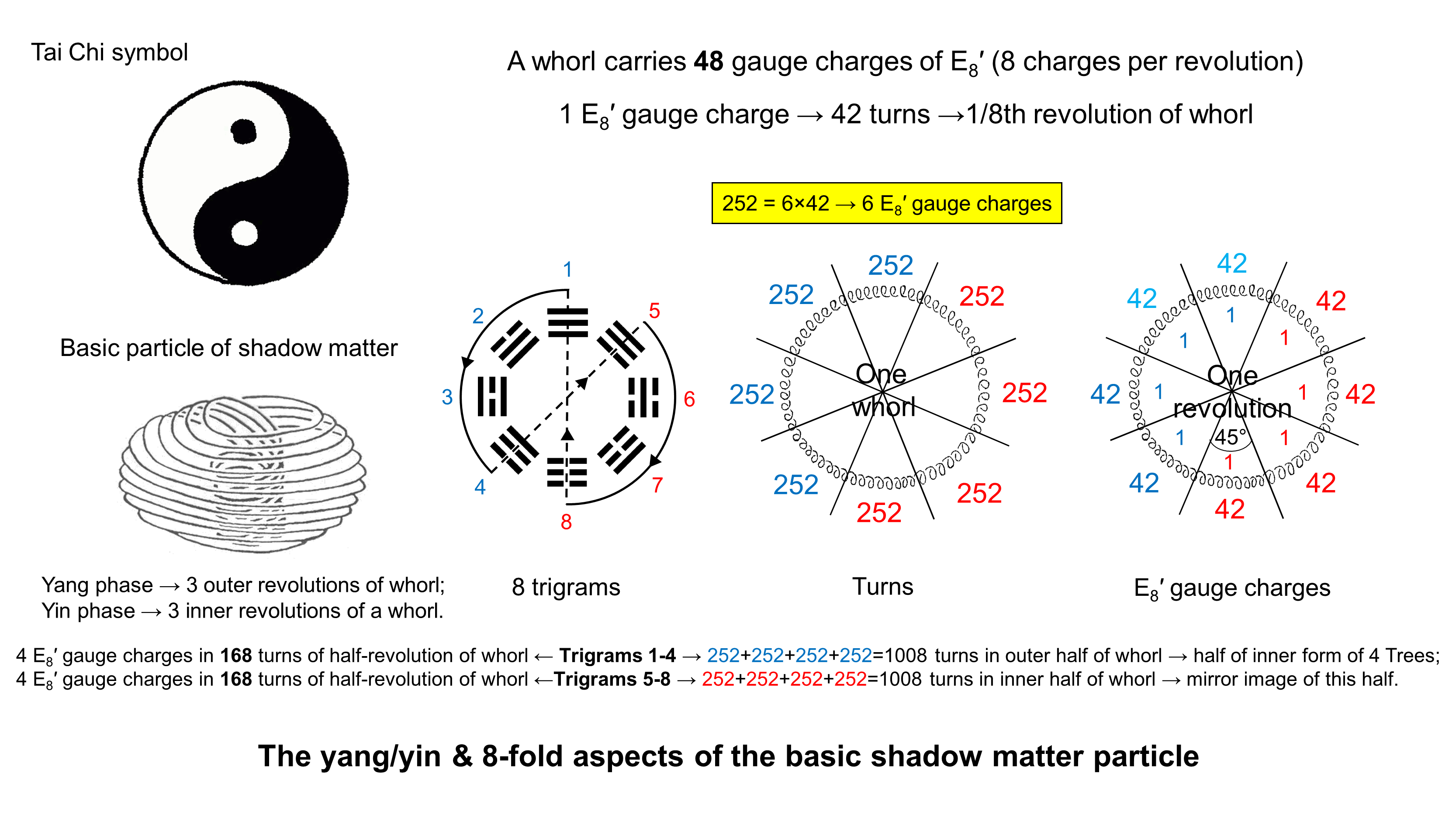The 240 gauge charges of E8′ are spread along the 30 revolutions of the five whorls of the basic particle of etheric matter, eight charges per revolution. Each whorl has to revolve six times around the spin axis of the particle because it carries 48 gauge charges, where 48 = 6×8. Starting with the Heaven trigram (number 1 in the diagram shown above), expressing maximum yang, the first four trigrams represent the diminishing-yang/increasing-yin phase of a cycle whose second half (yin phase) generates four trigrams whose lines and broken lines are the polar opposite of their diametric, but complementary opposite trigrams (1-8, 2-7, 3-6 & 4-5). Their counterparts in the basic particle of shadow/etheric matter are the eight E8′ gauge charges spread around one revolution of a whorl and the 252 turns carrying six charges in one-eight of a whorl that are symbolised by the 252 intrinsic yods outside root edges in each of the eight sets of seven enfolded polygons making up the inner form of the four uppermost Trees that map the four etheric subplanes. Trigrams 1-4 correspond to the three outer revolutions with 1008 turns (the expansive, yang phase of the complete cycle of revolution) and the trigrams 5-8 correspond to the three inner revolutions with 1008 turns (the yin phase). The outer and inner halves of each whorl correspond also to the four sets of seven polygons enfolded on one side of the central Pillar of Equilibrium of CTOL and to their mirror images on the other side (the two halves of the inner Tree of Life are its yang and yin aspects).

The top of the 7-tree is the 504th SL, counting from the apex of CTOL. The number value 168 of Cholem Yesodoth, the Mundane Chakra of Malkuth, specifies this point because it is the 168th SL on the Pillar of Equilibrium (denoted by yellow dots in the diagram below), whilst there are 168 SLs above it on each side pillar (coloured either indigo or violet), so that 504 = 3×168: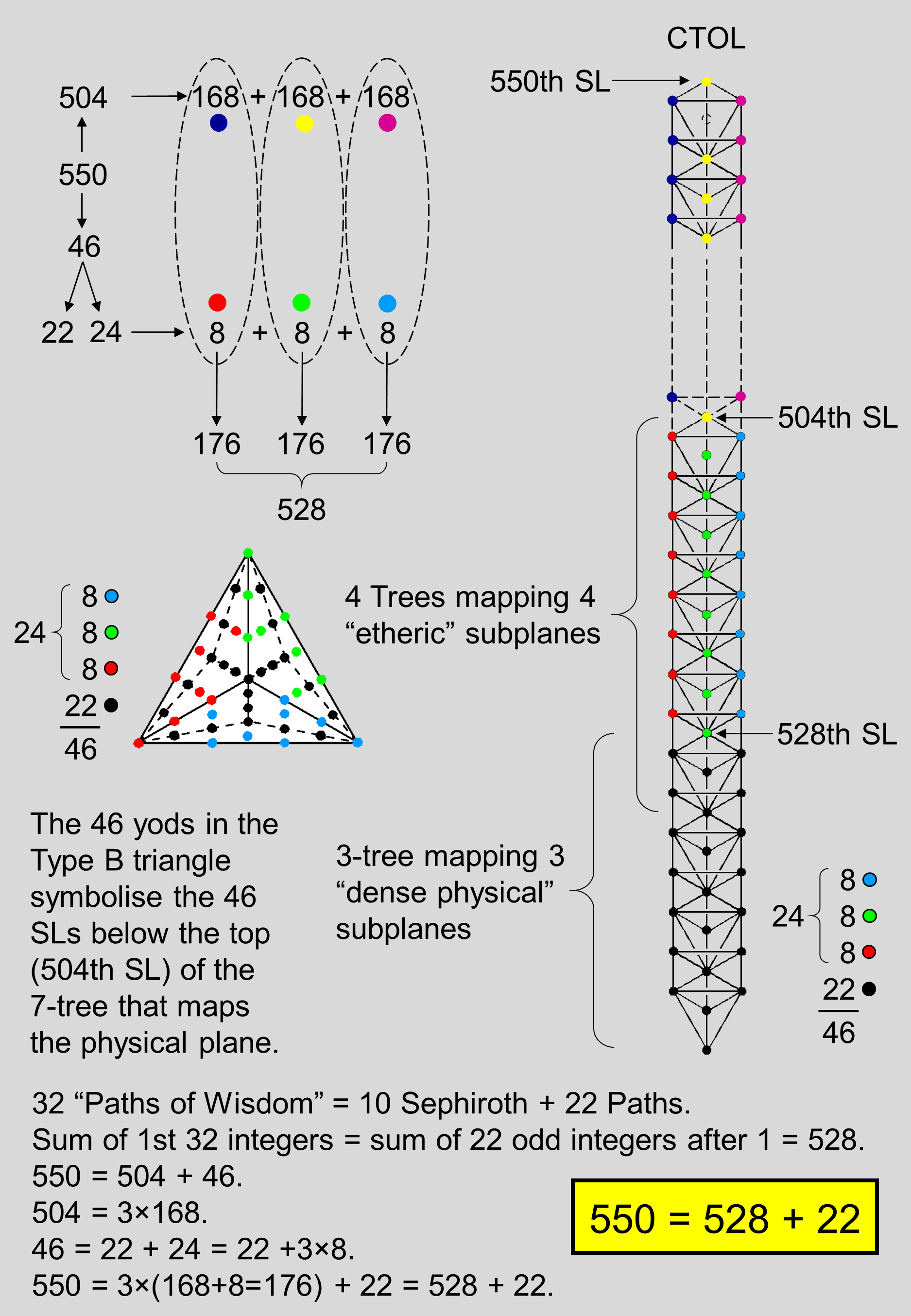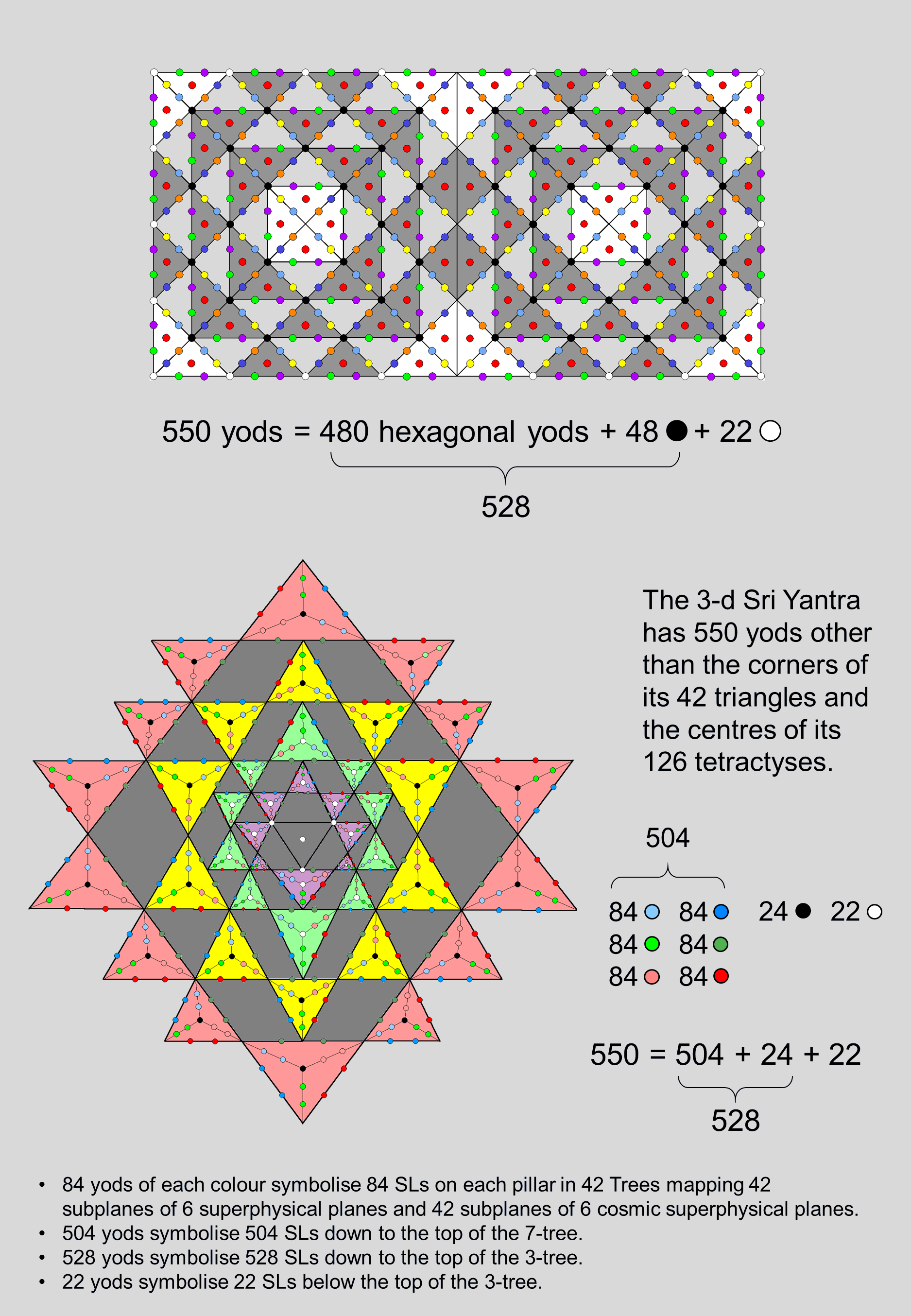Below the top of the 7-tree are 46 SLs, of which 24 SLs (eight per pillar, coloured either red, blue or green) extend down to the top of the 3-tree, below which are 22 more SLs (coloured black). This 22:24 division appears in the 46 yods of the Type B triangle. Three sets of eight yods (coloured tred, blue & green) either line sides of its sectors or are centres of tetractyses, leaving 22 black yods (21 surrounding the centre of the triangle) that line tetractyses inside each sector. It also appears in the 23 pairs of hexagonal yods that line the two joined dodecagons. 24 hexagonal yods line one dodecagon and 22 hexagonal yods line the other one. The 46 hexagonal yods symbolise the 46 chromosomes in the human cell, the pair of hexagonal yods on the root edge symbolising the X and Y sex chromosomes: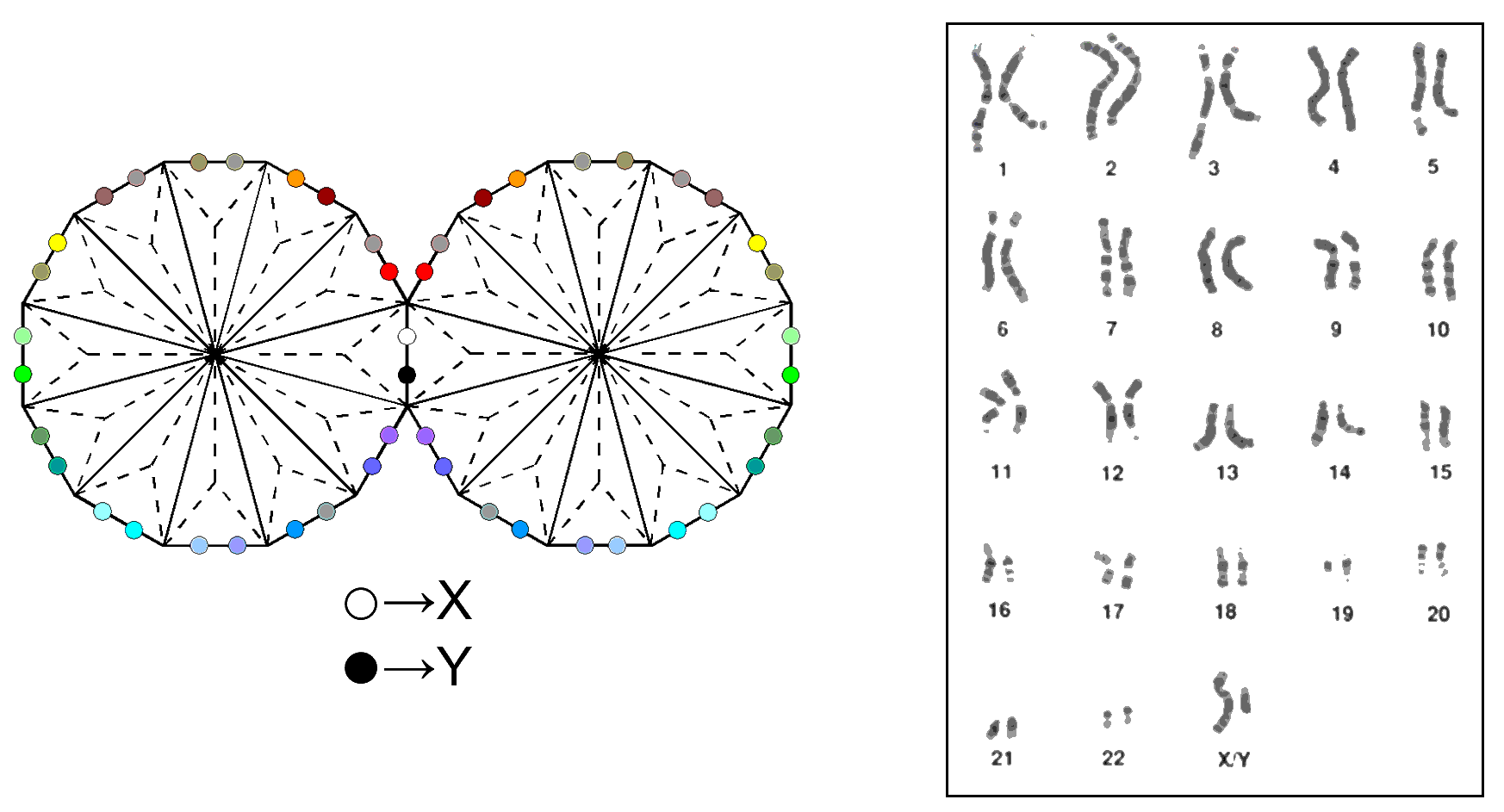It is remarkable that the number of SLs completing the 7-tree that maps the physical plane (the Malkuth level of the archetypal prototype of Adam Kadmon, or "Heavenly Man"), is the same as the number of chromosomes in the human cell. A chromosome is a long chain of DNA molecules that contain part or all of the genetic material of an organism. It is found in the nucleus of a cell, which normally contains 23 pairs of chromosomes (22 autosomes and a pair of gender-determining X and Y chromosomes, a human female having two X chromosomes and a male having one X and one Y chromosome). These 46 SLs consist of Chokmah & Binah of the 7th Tree (corresponding in this analogy to the pair of sex chromosomes X & Y), seven Chesed-Netzach pairs, seven Geburah-Hod pairs and eight Yesod-Malkuth pairs: 46 = 2 + 22×2. The Kabbalistic titles of "Abba" (Father) and "Alma" (Mother) given to Chokmah and Binah are consistent with the gender-determining function of the two sex chromosomes, whilst the 22 pairs of autosomes have their counterparts in the 22 pairs of Sephiroth lying on the three pillars of the 7-tree.

The 22:24 division appears also in the representation by the 3-d Sri Yantra of the 550 SLs of CTOL. When the 42 triangles surrounding the central one are Type A, 168 dark/light red hexagonal yods, 168 dark/light green hexagonal yods & 168 dark/light blue hexagonal yods line their 126 tetractyses. The three sets of 168 hexagonal yods correspond to the three sets of 168 SLs in CTOL down to the top of the 7-tree. The 22 white yods denoting the bindu, three corners of the central triangle and the centres of the 18 triangles in the first two layers correspond to the 22 lowest SLs in CTOL. The black yods at the centres of the 24 triangles in the last two layers correspond to the 24 SLs in the uppermost four Trees mapping the four etheric subplanes.

The number of yods surrounding the centre of a square whose sectors are 2nd-order tetractyses = 4×72 = 288. Surrounding the centres of two joined squares whose sectors are 2nd-order tetractyses are 550 yods outside their shared side, which has 13 yods:

288 + 288 − 13 − 13 = 550.

They symbolise the 550 SLs in CTOL. They comprise 480 coloured hexagonal yods, 48 internal, black corners of 80 1st-order tetractyses (24 black corners in each square) and 22 white corners on the sides of the two squares. Therefore,

550 = 480 + 24 + 24 + 22 = 504 + 24 + 22 = 504 + 46 = 528 + 22.

The 504:46, 24:22 and 528:22 divisions discussed above appear in this representation of CTOL.This should not be regarded as a coincidence, for the square is the symbol of the Tetrad and embodies in its construction from tetractyses or triangles all parameters of holistic systems.

The meaning of the number 528 vis-à-vis CTOL is that it is the number of SLs down to the top of the 3-tree, that is, it locates the commencement of the three Trees that represent what Theosophy refers to as the three "dense physical" subplanes wherein all material objects (as known to present-day physics) exist. They are the domain of the mineral, vegetable and animal (Including humans) kingdoms of nature. The number demarcates the universe perceptible to ordinary sensory awareness from all invisible levels of reality that only higher forms of consciousness can experience. They include the four classes of elementals and other denizens of the etheric world, such as some ghosts or earth-bound spirits. Although intangible and invisible, these entities exist in space-time but occupy a 10-d space-time sheet that is separated by a narrow gap along the tenth dimension of space required by M-theory. This is the universe of shadow matter, filled with far more matter than the universe known to astronomers and replete with its own life, although little about it has been established with any scientific rigor and what is known comes from folklore, mythology and parapsychology.

More evidence of the sacred connotations hidden in the number 528 is the fact that it is the thirty-second triangular number:

1 + 2 + 3 + ... + 32 = 528.

As it states in the opening line of the Sepher Yetzirah, the ten Sephiroth and the 22 letters of the Hebrew alphabet constitute "the 32 paths of mystical wisdom." It never occurs to students of Kabbalah to assign the first ten integers to the former and the next 22 integers to the 22 Paths of the Tree of Life according to the ordering of the letters that are associated with them: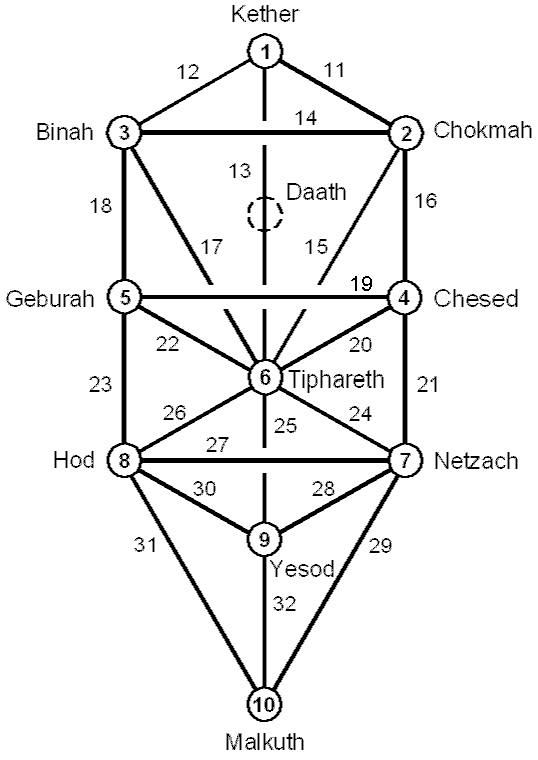Remarkably, the number 528 is also the sum of the first 22 odd integers after 1:

232 − 1 = 3 + 5 + 7 +... + 45 = 528.

As both the numbers 32 and 22 are key Kabbalistic numbers, this reveals the hidden, Kabbalistic significance of the number 528. However, its true meaning is more profound than these properties of simple arithmetic, as will now be explained. As

528  = 3 + 5 + 7 + 9 +... + 45 = (3+45) + (5+43) +... + (23+25)

= 48 + 48 +... + 48 = 11×48 = 5×48 + 6×48 = 240 + 288,

we see that the number 528 is the sum of 10 odd integers adding to 240 and 12 odd integers whose sum is 288, each number 48 being the sum of two odd integers. If the former are assigned to the 10 Paths present in the trunk of the Tree of Life and the latter are assigned to the 12 Paths making up its branches, this distinction between the two types of Paths generates the division of the number 528 into 240 and 288. As the seven enfolded, Type A polygons of the inner Tree of Life have 264 yods, their sides being lined by 120 yods and containing 144 yods, the two separate halves of the inner Tree of Life have (2×264=528) yods, of which (120+120=240) yods lie on the boundaries of the 14 enfolded polygons and (2×144=288) yods are inside them: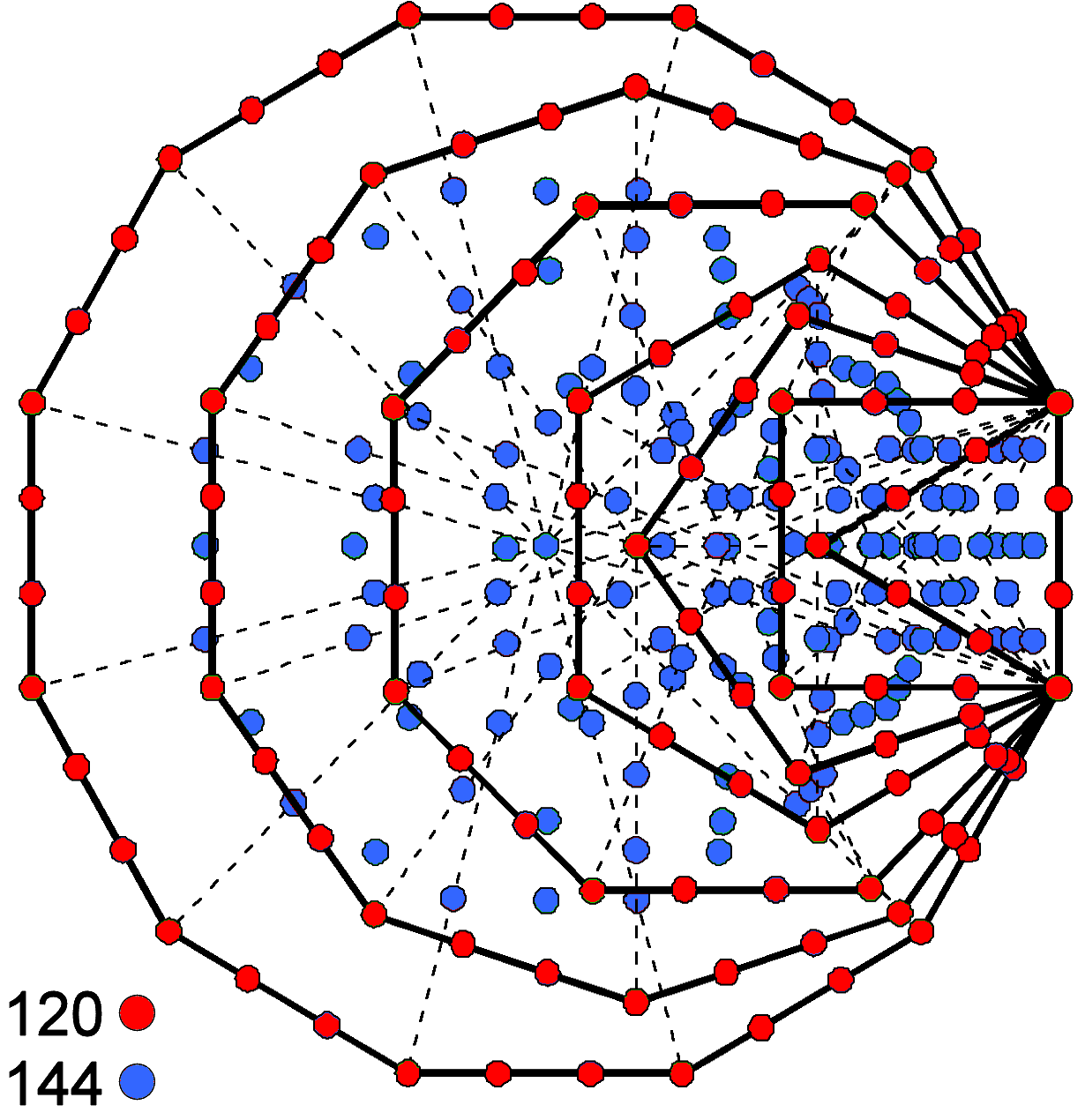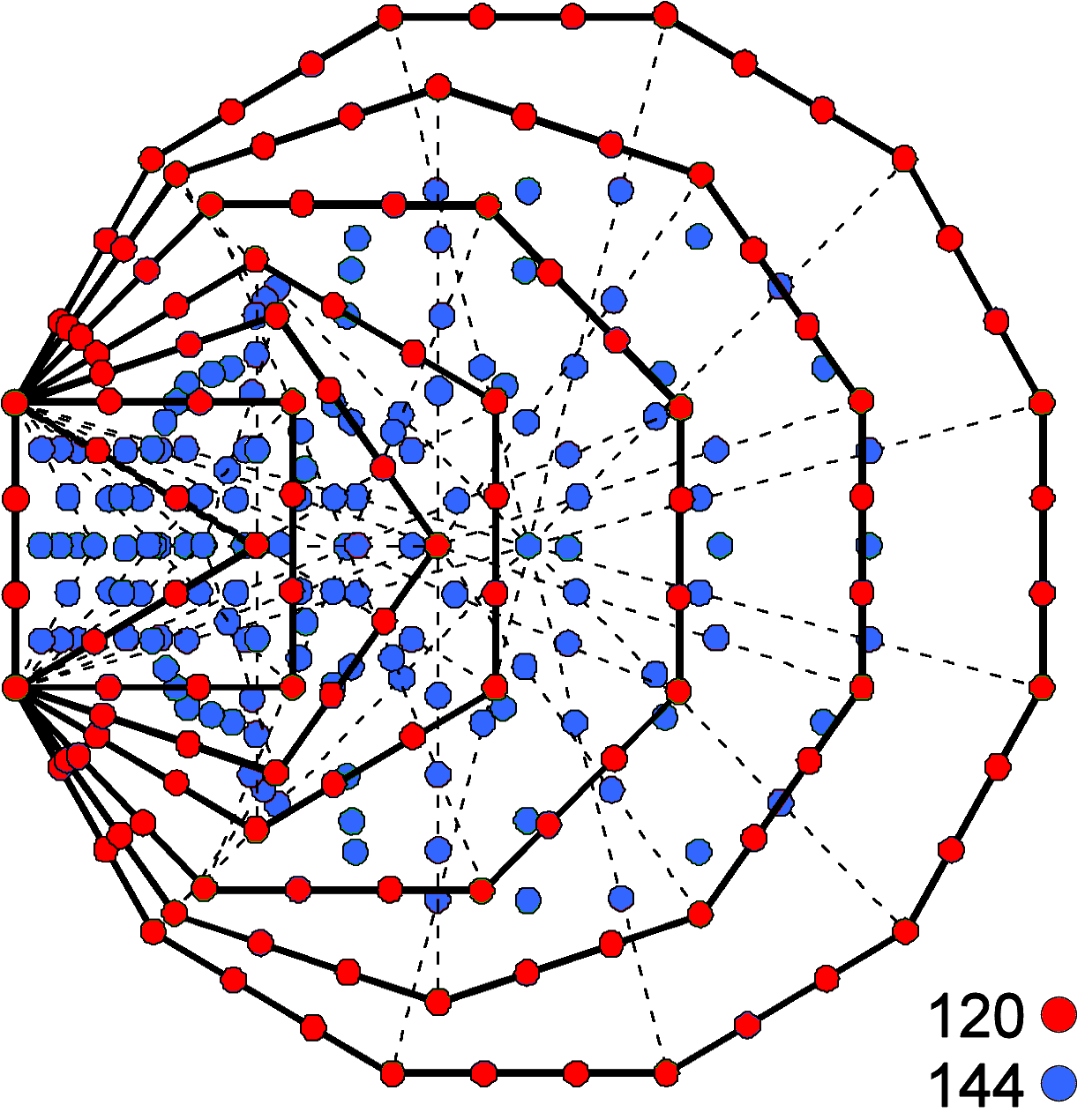The two separate halves of the inner Tree of Life contain 528 yods (240 boundary yods and 288 internal yods).

We see that the two types of yods (external & internal) making up the inner Tree of Life conform (in terms of their 5:6 proportion in numbers) to the basic distinction in its outer form between its trunk and branches — a distinction that moreover conforms to the arithmetic properties of the total yod population as the sum of the first 22 odd integers after 1. The same 10:12 division applies as well to each half of the inner Tree of Life because 120 is the sum of the first 10 odd integers after 1:

120 = 112 − 1 = 3 + 5 + 7 + 9 + 11 + 13 + 15 + 17 + 19 + 21,

and 144 is the sum of the first 12 odd integers:

144 = 122 = 1 + 3 + 5 + 7 + 9 + 11 + 13 + 15 + 17 + 19 + 21 + 23.

Another way in which the 240:288 division emerges in a natural, arithmetic way can be seen by noting that

172 − 1 = 288 = 3 + 5 + 7 + ... + 33,

so that 528 is the sum of the first 16 odd integers after 1, which add to 288, and the last six odd integers, which add to 240:

240 = 35 + 37 + 39 + 41 + 43 + 45.

Notice that the sum of the first two of these integers is 72 and the sum of the last four integers is 168. This compares with the 240 boundary yods in the 14 polygons comprising (36+36=72) corners and (84+84=168) hexagonal yods. The 72:168 division is found in the 240 roots of the exceptional Lie group E8 as the 72 roots of E6, one of its subgroups, and its 168 remaining roots. It manifests in the subquark state of the E8×E8′ heterotic superstring as the 72 E8 gauge charges of E6 that are spread along the three major whorls of the UPA, 24 per whorl, and as the 168 other gauge charges of E8 that are spread along its seven minor whorls, 24 per whorl.

The 47 tetractyses that make up each set of seven enfolded Type A polygons comprise 41 corners, 47 central hexagonal yods (i.e., 88 corners & centres) and 88 pairs of hexagonal yods lining their 88 sides. This means that the 264 yods in each separate half of the inner Tree of Life comprise three sets of 88 yods: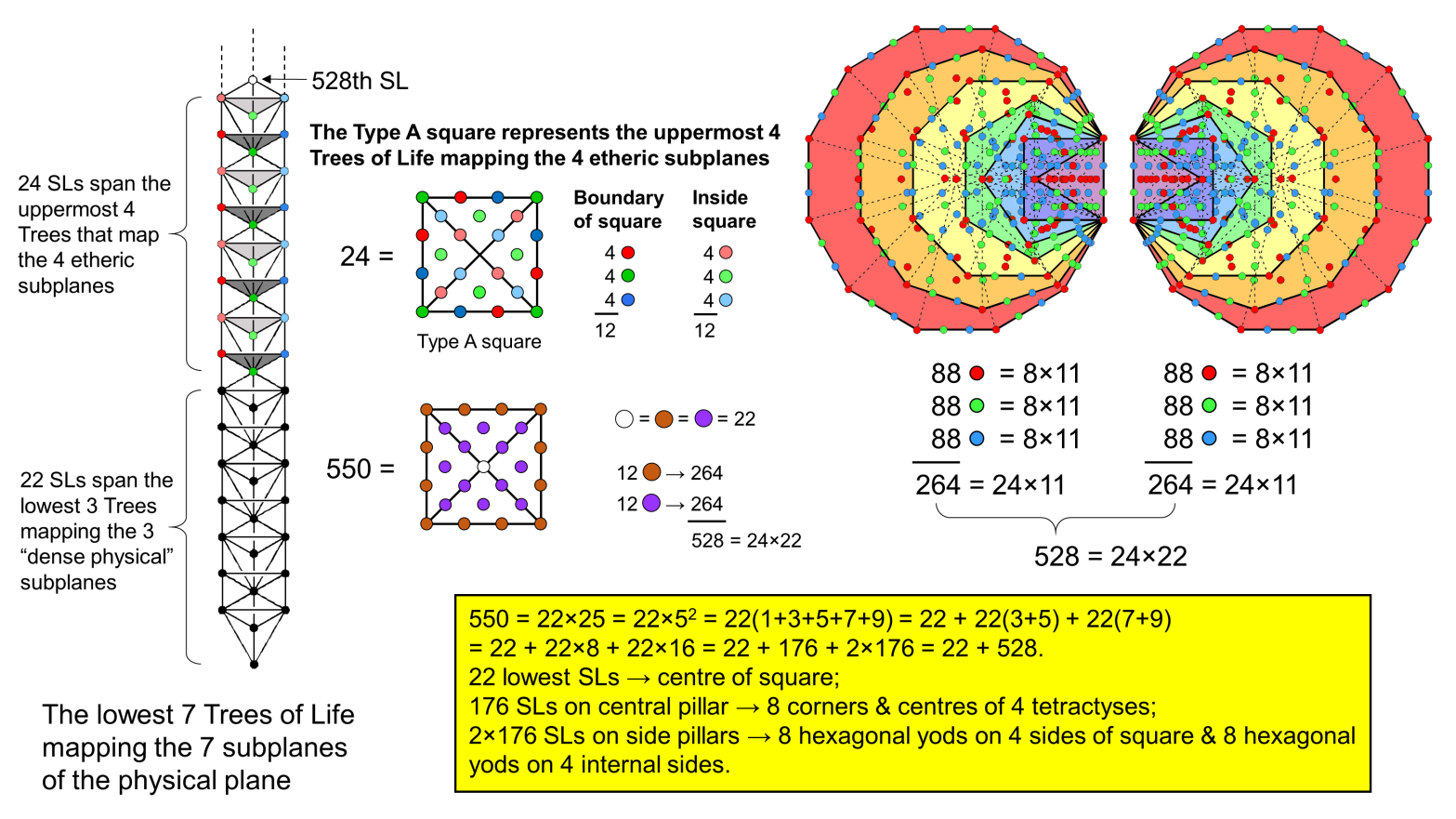The factorisation of 88 as 8×11 means that there are (264×2=528) yods in both halves, where 528 factorises as 24×11×2, i.e., 24×22. A Type A square has 24 yods surrounding its centre. Weighted with the Kabbalistic number 22, it represents the number 528. Assigning the same weight to its centre, it represents the (22+528=550) SLs in CTOL. The central yod denotes the lowest 22 SLs up to Chokmah of the 3rd Tree and the 24 yods surrounding it denote the 528 SLs in the 88 Trees above the 3-tree. The 12 brown boundary yods denote the 264 yods in one set of seven enfolded polygons and the 12 internal violet yods denote the 264 yods in the other set. The four corners denote the 88 corners & central hexagonal yods in one set and the four pairs of brown hexagonal yods denote its 88 pairs of hexagonal yods. The four violet hexagonal yods at centres of tetractyses denote the 88 corners & central hexagonal yods in the other set, whilst the four pairs of violet hexagonal yods denote its 88 pairs of hexagonal yods.

The 24 yods surrounding the centre of the Type A square also symbolise the 24 SLs in the four uppermost Trees of the 7-tree that maps the physical plane. The four dark green corners and four light green central hexagonal yods correspond to the four pairs of SLs on the central pillar in the four Trees. The four dark/light red hexagonal yods correspond to Binah & Geburah in each Tree and the four dark/light blue hexagonal yods correspond to Chokmah & Chesed in each Tree. As 22 = 1 + 21, assigning the number value 21 of EHYEH, the Godname of Kether, to each yod surrounding the centre of the Type A square generates the number 504 (=24×21) as the number of SLs down to the top of the 7-tree. The number 21 is the least number of yods needed to delineate the four sectors of a Type A square constructed from four tetractyses: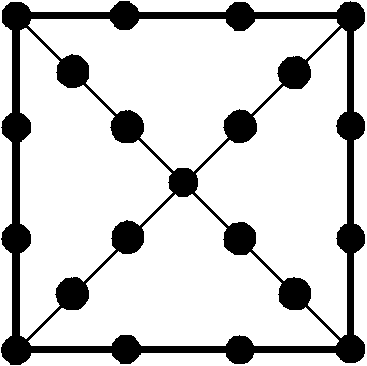This simple splitting of the Kabbalistic number 22 generates through the vehicle of the square two very important parameters of the spiritual cosmos: 504 and 528. The Type A square is the 2-d projection of a square pyramid whose four faces are tetractyses. This well-known shape has been attributed with many properties (some real, some not so). Here are two more of the former type: it embodies two numbers that demarcate the physical universe from all superphysical levels of reality. In fact, as 550 = 25×22, it is a representation of the 550 SLs in CTOL itself when each yod is weighted with the number 22. As has been discussed in previous pages of this section, the 25 yods in the Type A square symbolise the 25 spatial dimensions of the 26-d space-time predicted by quantum mechanics for spinless (bosonic) strings. Its central yod denotes their large-scale, longitudinal dimension and the 24 yods surrounding it symbolise their 24 transverse dimensions. The four corners and the four central, hexagonal yods form a set of eight yods, and the 16 hexagonal yods on sides form two sets of eight, all three sets of eight yods being analogous to the eight trigrams and to the three sets of eight SLs in the four uppermost Trees mapping the four etheric subplanes discussed earlier. This 8:16 division of yods in the square has its counterpart in E8×E8′ heterotic superstring theory as the eight transverse dimensions of 10-d superstring space-time and the 16 higher dimensions of 26-d space-time containing the bosonic modes of oscillation. It is a characteristic pattern of all holistic systems and their sacred-geometrical representations, for they are but different versions of one archetypal system. An example of this pattern in a holistic system is the 24-cell. Its 24 vertices consist of the eight vertices of the 16-cell and 16 vertices that form an 8-cell (see here). As

24 = 52 − 1 = (3 + 5) + (7 + 9) = 8 + 16,

the pattern expresses the arithmetic properties of the first four odd integers after 1, the Monad. For the ancient Pythagoreans, this was the source and principle of all numbers.

The geometry of the outer & inner forms of the Tree of Life embodies the number 528

We shall now prove that the cosmic parameter 528 quantifies the geometrical composition of the outer and inner forms of the Tree of Life. In other words, every single geometrical element that constructs them has its counterpart in the 528 SLs of the 88 Trees of Life mapping the 88 subplanes above the three subplanes that are the domain of the mineral, vegetable & animal kingdoms of nature.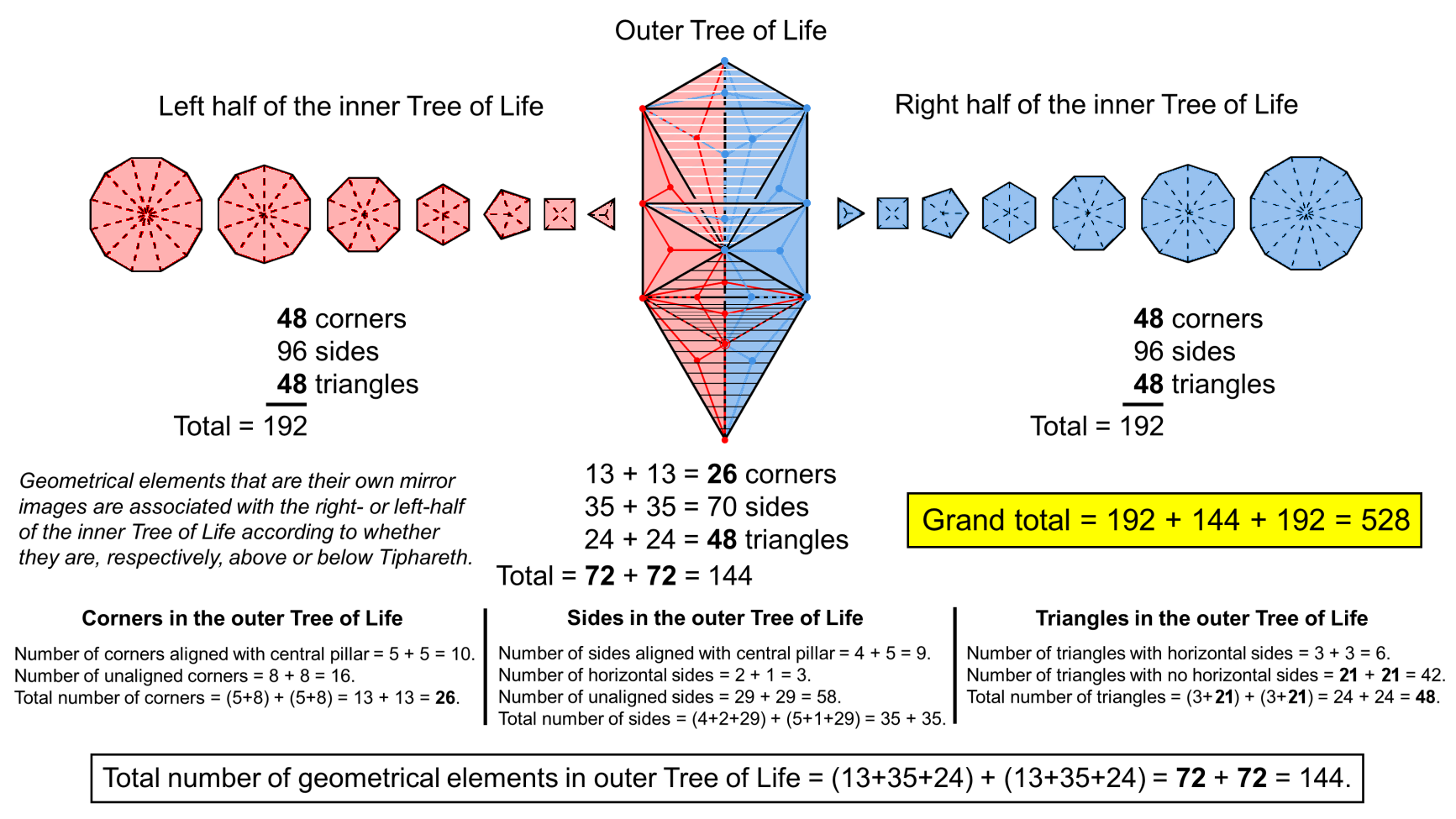528 points, lines & triangles shape the outer & inner forms of the Tree of Life.

The number of points (corners), lines (sides) & triangles (sectors) making up the Type A n-gon = 4n + 1, where "1" denotes its centre. The seven Type A polygons with 48 corners in each half of the inner Tree of Life have (4×48=192) geometrical elements surrounding their centres. As points, the latter play no part in shaping each polygon — only the 192 geometrical elements perform this task — and therefore there is no need to include them in an analysis of what geometrical elements create the form of the outer & inner Trees of Life. For this reason, whenever the terms "geometrical composition" or "total number of geometrical elements" are used, it will refer not to the mathematically complete set of geometrical elements but to those surrounding centres, whenever polygons are included, that, effectively, determine their form. The total number of geometrical elements shaping the two halves of the inner Tree of Life = 192 + 192 = 384, where

384 = 22 + 32 + 42 + 52 + 62 + 72 + 82 + 92 + 102.

384 = 96 corners + 192 sides + 96 triangles = 192 corners & triangles + 192 sides.

The parameter 384 divides into two equal halves in two ways. The outer Tree of Life consists of 16 triangles with 10 corners and 22 sides (48 geometrical elements). When each is a Type A triangle, it has one corner and three internal sides of three sectors, i.e., seven geometrical elements. This means that the conversion: triangle→Type A triangle adds seven internal geometrical elements, so that the number of geometrical elements in the 16 Type A triangles of the outer Tree of Life = 10 + 22 + 16×7 = 144 (26 corners, 70 sides & 48 triangles). As 144 = 48×3, this is also the number of corners & sides (or sides & triangles) in each set of seven separate Type A polygons. The total number of geometrical elements in the outer & inner Trees of Life = 144 + 384 = 528. Remarkably, this is — as we have discussed — the number of SLs in CTOL down to, and including, the top of the 3-tree.

The geometrical composition of both the outer & inner Trees = (96+26=122) corners + (192+70=262) sides + (96+48=144) triangles. The number of corners & triangles is two more than 264 and the number of sides is two less than this number. Therefore, the geometrical composition does not divide neatly into two sets of 264, as shown by the yod composition of each set of seven enfolded polygons: 528 = 264 + 264. However, in view of the fact that each set of polygons has the same composition and the fact that the outer Tree of Life has axial, or left-right symmetry, it would seem possible, intuitively speaking, to define two "halves" of the latter in some natural way so that there are 264 geometrical elements in the left half of the combination of Trees and 264 geometrical elements in the right half. The issue of whether this is actually possible is complicated by the fact that some points, lines & triangles are either aligned with, or straddle, the central Pillar of Equilibrium, meaning that they are their own images when reflected in a mirror that contains this pillar, which is not a straight line but bent, although only in one dimension, so that it still lies in a plane that is perpendicular to the plane containing the straight Pillars of Mercy and Severity. As the total number (144) of its geometrical elements is an even integer, there must be an even number of geometrical elements that are their own images. This means that it should be possible for them to form two groups, with the same number associated with the left side as that associated with the right side. As it is in principle possible, this partitioning could be done in merely a formal way that disregarded the actual geometry. But it would be more satisfying to identify a partition that naturally fitted it. We have to determine which mirror-image geometrical elements can be associated with those on the the left of the central Pillar of Equilibrium and which similar ones can be associated with the right side. At the bottom of the picture shown above are listed the numbers of unaligned and aligned corners, sides & triangles in the outer Tree of Life. The five red triangles are the mirror images of their blue triangle counterparts. This leaves six triangles with corners at:

 Kether-Chokmah-Binah Tiphareth-Netzach-Hod Binah-Chokmah-Tiphareth Hod-Netzach-Yesod Geburah-Chesed-Tiphareth Hod-Netzach-Malkuth

that contain some geometrical elements, each of which is its own mirror image when reflected across the central axis of symmetry. Three of these triangles (to be associated with the five blue triangles) are shaded with white, horizontal lines and three (to be associated with the five red triangles) are shaded with black, horizontal lines. Tiphareth, the centre of the outer Tree of Life, metaphysically speaking, is the point of partition that separates the 16 triangles into two sets of eight triangles — the two "halves" of the outer Tree of Life. The pairs of numbers indicated in each line at the bottom of the diagram for the geometrical composition of the outer Tree refer to these two sets of triangles. Notice in particular that the 26 corners of the 48 triangles consist of 10 corners aligned with the central pillar and eight corners on either side. The former includes four Sephirothic points, creating the division:

26 = 8 + 10 (=4+6) + 8 = 4 + 6 + 16.

Remarkably, this is identical to the pattern of dimensions in the 26-d space-time of bosonic strings. The number "4" is the number of dimensions of Minkowski space-time that Einstein worked with in his Special Theory of Relativity, the number "6" is the number of compactified dimensions in 10-d superstring space-time and the number "16" is the extra number of higher dimensions of the space-time of bosonic strings. The 4:6 distinction in superstring dimensions corrresponds here to the difference between the four aligned corners that belong to the original Tree of Life because they are Sephirothic points and the six corners generated by transforming the 16 triangles into Type A triangles. The number of each type of geometrical element is the same for either, now-defined half of the outer Tree of Life; this is what is required. It means that the outer Tree of Life can be divided into two sets of eight triangles — two halves — with the same numbers of each type of geometrical element. Each half with 72 geometrical elements can then be associated with a set of seven Type A polygons to create two sets of 264 geometrical elements that parallel the two halves of the inner Tree of Life with 264 yods. Each set consists of 61 corners, 131 sides & 72 triangles. The number 72 is the gematria number value of Chesed and the number 131 is the number value of Samael, the Archangel of Geburah (see here). The following tetractys array of squares of integers generates as their sum the number 528: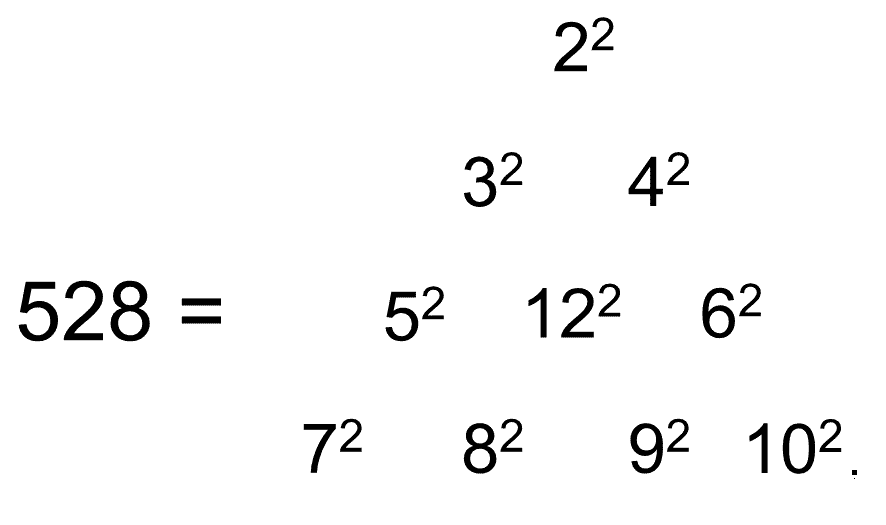The sum of the squares of the nine integers 2-10 on its boundary is 384. This is the number of corners & sides of the 144 triangles in the combined outer & inner Trees of Life, where 144 is the square 122 at the centre of the tetractys.

We discovered earlier that each set of seven enfolded Type A polygons contain 264 yods that form three sets of 88 yods: 88 corners & centres of their 47 tetractyses and two sets of 88 hexagonal yods that line their 88 sides. The 528 yods in both sets of polygons consist of three sets of (88+88=176) yods. Does the factorisation 3×176 manifest in the 528 geometrical elements making up the outer Tree of Life and the 14 separate polygons of its inner form? Yes, as we shall now show. The six polygons other than the hexagon in each set of seven Type A polygons have 42 sectors with (42×4=168) geometrical elements surrounding their centres. Each set is associated with the eight Type A triangles that are in each "half" of the outer Tree of Life. Grouping their centres as corners of sectors with each set of 168 geometrical elements creates two sets, each with (42+8=50) corners and 176 geometrical elements, where 50 is the number value of ELOHIM, the Godname of Binah. The outer Tree of Life has 144 geometrical elements (128 other than these 16 centres). The number of geometrical elements in the two hexagons and the outer Tree of Life other than these centres = 128 + 24 + 24 = 176. The outer & inner Trees of Life therefore have 528 geometrical elements that naturally form three sets of 176. They have not been randomly picked to create these sets, for that would always be possible, and any such selection would have no significance. Instead, the three sets have been shown to exist by combining well-defined groups of geometrical elements, each group referring to one object. The three sets of 176 geometrical elements correspond to the 176 SLs on each side pillar of CTOL above the 3-tree and to the 176 SLs on the central pillar, including the apex of the 3-tree. The geometrical composition of the outer & inner Trees is isomorphic to the 88 Trees, each with two SLs per pillar, that map all levels of reality beyond the physical universe mapped by the 3-tree.

The outer & inner forms of the Tree of Life embody the number 528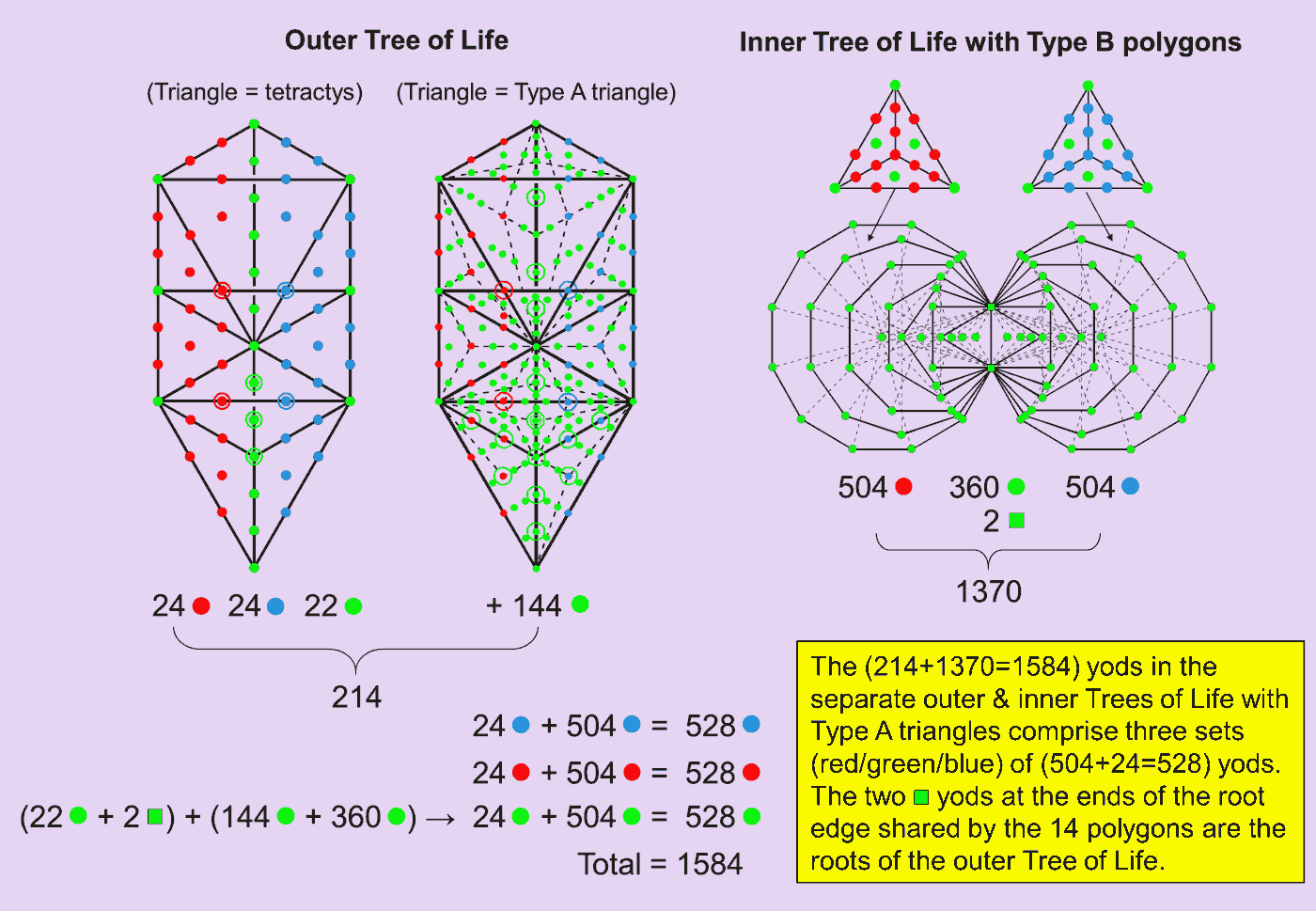When its 16 triangles are tetractyses, the outer Tree of Life has 70 yods. They comprise 10 green Sephirothic corners, 12 more green yods aligned with the central Pillar of Equilibrium, 24 red yods on its left side and 24 blue yods on its right side. When its triangles are Type A, nine more green yods are added to the interior of each one. This means that the number of yods in the outer Tree of Life with Type A triangles = 70 + 16×9 = 214. When the 14 polygons in the inner Tree of Life are Type B, they have 1370 yods. The sum of the yod populations of the outer & inner forms of the Tree of Life is 1584. This is 3×528. We have seen that the number 528 is the sum of the yod populations of each separate half of the inner Tree of Life. Let us see whether this factorisation has a simple basis in terms of the way in which the yods of the separate outer and inner Trees are distributed.

The 14 polygons have 94 sectors with 80 green corners. A Type A triangle has three green hexagonal yods at the centres of its sectors. When the polygonal sectors are Type A triangles, they have (3×94=282) of this type of yod, where 282 is the number value of Aralim ("Thrones"), the Order of Angels associated with Binah. The 282 tetractyses in the 14 Type B polygons contain (80+282=362) green yods that are either their corners or centres. Two of them are the endpoints of the root edge shared by the polygons. The number of remaining yods that line the sides of tetractyses = 1370 − 362 = 1008. Associated with each half of th‌e Tree of Life are (1008/2=504) such yods; they are coloured either red or blue in the diagram shown above.

The 24 blue yods and the 24 red yods in the outer Tree of Life form pairs that are mirror images of each other when reflected in a mirror perpendicular to the plane formed by the two side pillars and containing the central pillar. Similarly, the 504 blue yods in the right half of the inner Tree of Life are the mirror images of the 504 red yods in its left half. Hence, the (24+504=528) red yods in one half have their blue mirror image counterparts in the other half. The 22 green yods aligned with the central pillar are their own mirror images. Associating them with the two green end points of the root edge creates a set of 24 yods which, when grouped with the 360 green corners & centres of tetractyses and the 144 green yods added by transforming the 16 triangles of the outer Tree into Type A triangles, creates a set of 528 green yods. The 1584 yods in the separate outer and inner Trees form three distinct sets of 528 yods.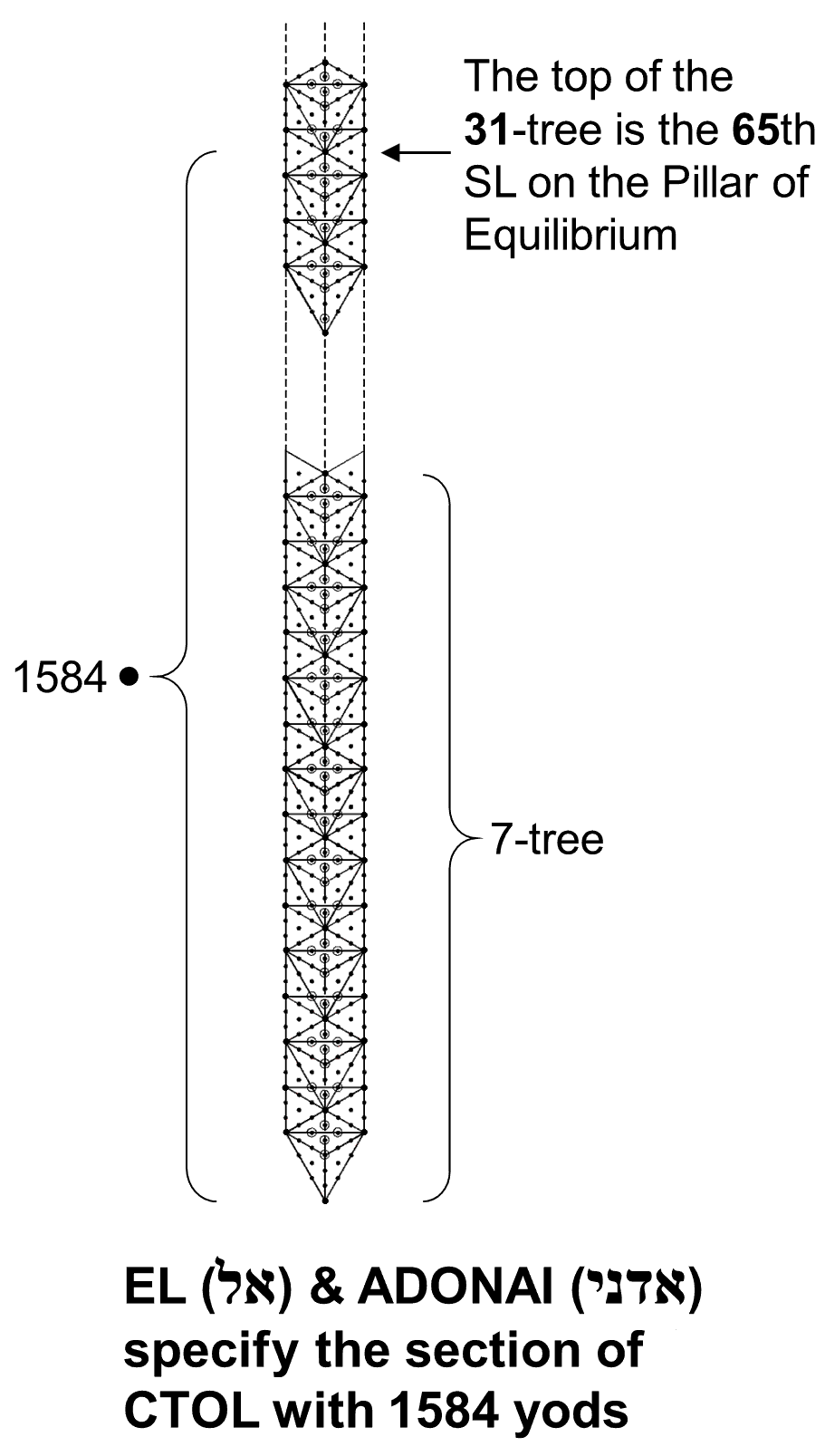It is, of course, always possible to create three groups of yods, each with 528 yods randomly picked from a set of 1528 yods. But this is not a sensible reason for dismissing the property just derived as lacking significance, for it wrongly characterises the calculation. Firstly, the 528 yods in each group are not arbitrarily selected; they are assembled from complete sets of various classes of yods, or combinations thereof. The point is worth making that it is not trivial that such an assembly should turn out to be even possible. Secondly, each group is made up of a set of 24 yods and a set of 504 yods. Previous derivations of the holistic parameter 528 in various sacred-geometrical contexts showed how it exhibits being compounded from a set of 24 elements and a set of 504 elements. In the context of the 528 SLs in CTOL down to the top of the 3-tree, there are 24 SLs above it as far as the top of the 7-tree, which is the 504th SL. Those 24 SLs span the four Trees mapping the four, so-called "etheric subplanes" of the physical plane. The factorisation 1584 = 3×528 is only made possible by the non-trivial fact that the Tree of Life with 70 yods and its root of two yods can form three natural sets of 24, namely, two sets of 24 that are paired as mirror images of each other and a third set of 24 yods, each of which is its own mirror image. Obviously, the yods in each of these three sets are not arbitrarily selected, for they either make up the left-hand or right-hand sides of the outer Tree or are aligned with its central pillar; similarly for the set of 504 yods intrinsic to each half of the inner Tree of Life. Either the triple appearance of the number 528 is due to miraculous chance (a possibility which we reject as very implausible) or it indicates some highly significant meaning for the number 1584. As it also factorises as 22×72, assigning the number value 72 of Chesed to each of the 22 Paths of the Tree of Life generates this number. In the context of CTOL, there are 1584 yods up to the level of the top of the 31st Tree, which is the 65th SL on the central Pillar of Equilibrium. The Godname EL ("God") of Chesed with number value 31 marks out a section of CTOL that comprises 1584 yods; it is marked out also by the Godname ADONAI of Malkuth with number value 65.

The significance for superstring physics of the 31-tree is that, being the 65th SL on the Pillar of Equilibrium, its apex is marked out by the Godname of Malkuth because it is Malkuth of the 33rd Tree of Life, so that, as the number of yods in the n-tree is

Y(n) = 50n + 30,

Y(33) = 1680. In other words, the yod population of the 33-tree is exactly the number of turns counted by C.W. Leadbeater in each helical whorl of the UPA. It can be claimed that this is the true reason** why the masonic number 33 is central to the mathematical description of the physical universe: through its yod population, this number of Trees, alone, determines the oscillatory form of the subquark state of E8×E8′ heterotic superstrings as the subatomic manifestation of the Tree of Life blueprint. This unique property of the 33-tree needs to be properly understood in the wider context of the 49-tree that maps the cosmic physical plane. The 49-tree contains Y(49) = 2480 (=248×10) yods. There are 800 (=80×10) yods above the 33-tree, which contains 1680 (=168×10) yods. Compare this division with the fact that the 248 roots of E8 comprise its eight simple roots, the 72 roots of E6 and 168 roots other than these 80 roots. E8-symmetric superstring forces are mediated in 10-d space-time by the virtual exchange of 248 gauge fields, each being a vector field with 10 components. Every yod in the 49-tree that maps the "cosmic Malkuth," namely, the cosmic physical plane, symbolises one of these components. The 49-tree embodies the dimension of E8. The 1680 yods in the 33-tree prescribed by ADONAI denote the 1680 components of the 168 vector gauge fields that couple to charges defined by the 168 roots of E8 that are neither its simple roots nor roots of its exceptional subgroup E6. The 72:168 division of roots manifests in the UPA as the 72 E8 gauge charges carried by its three major whorls (24 charges per whorl) and the 168 E8 gauge charges that are spread along its seven minor whorls (24 charges per whorl). The structural difference between these whorls is due to symmetry breaking of E8 into E6, which introduces a slightly different winding number for the major whorls, every 25 nth-order spirillae being composed of 176 (n+1)th-order spirillae, not 175, as is the case for the minor whorls.*** Cowen did not notice any obvious difference between the five whorls of the shadow matter particle. Whether there are differences but they were too subtle to be detected by a superficial examination (Cowen never checked for this) is an issue that future investigations must settle. Whether every whorl in this particle comprises the same number of turns also needs checking (no trivial task, given that each one is predicted to have 2016 turns!). It should have this property because:

1. Cowen reported that every whorl revolves the same number of times around the spin axis of the particle;
2. the number of turns in each revolution of a whorl of the shadow matter particle is expected to be the same for all its whorls, just as it is for the UPA; this is predicted to be 336 (the value for the UPA) because the ratio of the numbers of turns in a whorl of each type of particle is then, simply, the ratio of the numbers of their revolutions, namely, 6/5, as required by the analogy discussed in previous pages (e.g., page 4) between the two polyhedra that make up the Polyhedral Tree of Life, as well as that between the trunk and branches of the outer Tree of Life.

* Proof: the number of yods in the n-tree composed of tetractyses ≡ Y(n) = 50n + 30. Hence, Y(31) = 1580. There are two yods up to its apex outside the 31-tree on either side of the central pillar (see the accompanying picture). The number of yods up to the level of the apex = 1580 + 2 + 2 = 1584.
** The 33rd Tree maps the fifth subplane of the fifth plane because 33 = 4×7 + 5. As the seven planes of consciousness denote the domains of the seven Sephiroth of Construction, the fifth (Atmic) plane corresponds to Tiphareth, the fifth Sephirah of Construction, as does its fifth subplane. The 33rd Tree is therefore unique in that its Tiphareth is that of the Tree mapping the subplane that most expresses the quality of Tiphareth.
*** The counterpart in the inner Tree of Life of this (175→176) augmentation exists because the first six enfolded polygons have 26 corners, one of which is shared with polygons in the inner form of the next higher Tree, so that they have 25 intrinsic corners per Tree, i.e., the first six types of polygons enfolded in seven overlapping Trees have 175 intrinsic corners and 176 corners in total, one being shared with polygons in the inner form of the next higher Tree. It appears also in the geometry of each half of the inner Tree, for the seven enfolded Type A polygons have 176 corners, sides & triangles, of which the topmost corner of the hexagon coincides with the lowest corner of the hexagon belonging to the inner form of the next higher Tree, leaving 175 geometrical elements that are intrinsic to the polygons in the inner form of each Tree.

 << Previous    1...   13  14    16  17  ...19    Next >>

Home